# Exponent Worksheets

#### What are Exponents?

In mathematics, exponents are the contracted form of larger value of multiplication. For example, three multiply by six time is equal to 729 (3 × 3 × 3 × 3 × 3 × 3 = 729) so this multiplication method is long. We do not enough time to solve such lengthy value that is why we need short cut. So, mathematician take leverage of exponents. There are two categories to learn exponents in numbers that is power and base. The second form or name of exponent is POWER that we express it in short form. For instance, if we have a number 3 and another value expressed in a small form at the above side of 6 then, we will consider a digit three Base and the digit six Power. There are also other forms of exponent that mathematicians use in the questions. That are Negative and Positive exponents. There are different methods to solve both of them. When we have negative exponents, we will remove the sign of subtraction by converting the bases into division or fraction form. For instance, if we have the Base 7 and Power -2 like this 7-2, we will convert the value into fraction form like this 1/7.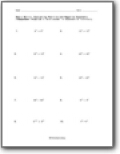###### Evaluating Positive and Negative Exponents

Give answer in exponential form only.This is a more advanced version of the previous set of worksheets. Example: (288 x 2812 x 285) ÷ 2811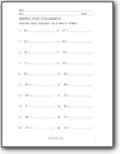###### Simplifying Exponents

Express each exponent as a whole number. Some are easy, some are complex.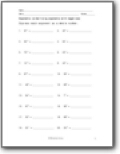###### Simplifying Negative Exponents

Express each exponent as a whole number.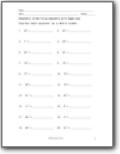###### More Negative Practice

This is a skill that eludes many students, so we give you more work with it.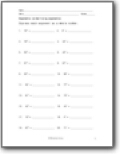###### Make Whole Numbers

Express each exponent as a whole number.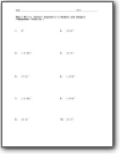###### Convert Exponents to Numbers and Compare

We include both decimals and fractions in this set.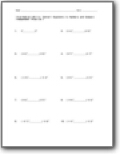###### Comparing Exponential Values

You will need to place a symbol to compare the values that are presented.